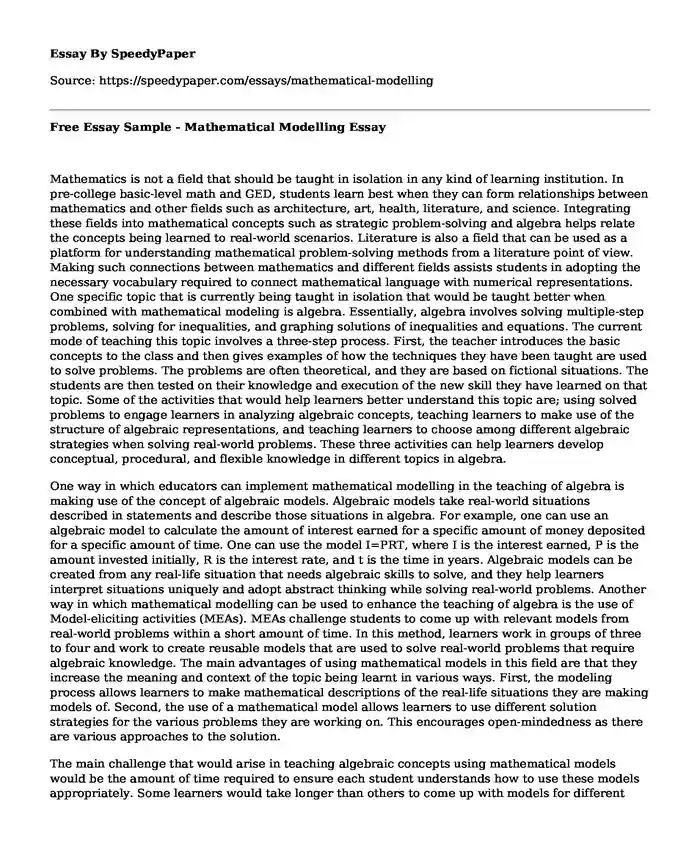# Free Essay Sample - Mathematical Modelling

Published: 2023-09-24Essay type: Quantitative research papers Categories: Learning Knowledge Students Mathematics Pages: 3 Wordcount: 695 words
143 views

Mathematics is not a field that should be taught in isolation in any kind of learning institution. In pre-college basic-level math and GED, students learn best when they can form relationships between mathematics and other fields such as architecture, art, health, literature, and science. Integrating these fields into mathematical concepts such as strategic problem-solving and algebra helps relate the concepts being learned to real-world scenarios. Literature is also a field that can be used as a platform for understanding mathematical problem-solving methods from a literature point of view. Making such connections between mathematics and different fields assists students in adopting the necessary vocabulary required to connect mathematical language with numerical representations. One specific topic that is currently being taught in isolation that would be taught better when combined with mathematical modeling is algebra. Essentially, algebra involves solving multiple-step problems, solving for inequalities, and graphing solutions of inequalities and equations. The current mode of teaching this topic involves a three-step process. First, the teacher introduces the basic concepts to the class and then gives examples of how the techniques they have been taught are used to solve problems. The problems are often theoretical, and they are based on fictional situations. The students are then tested on their knowledge and execution of the new skill they have learned on that topic. Some of the activities that would help learners better understand this topic are; using solved problems to engage learners in analyzing algebraic concepts, teaching learners to make use of the structure of algebraic representations, and teaching learners to choose among different algebraic strategies when solving real-world problems. These three activities can help learners develop conceptual, procedural, and flexible knowledge in different topics in algebra.Is your time best spent reading someone else’s essay? Get a 100% original essay FROM A CERTIFIED WRITER!

One way in which educators can implement mathematical modelling in the teaching of algebra is making use of the concept of algebraic models. Algebraic models take real-world situations described in statements and describe those situations in algebra. For example, one can use an algebraic model to calculate the amount of interest earned for a specific amount of money deposited for a specific amount of time. One can use the model I=PRT, where I is the interest earned, P is the amount invested initially, R is the interest rate, and t is the time in years. Algebraic models can be created from any real-life situation that needs algebraic skills to solve, and they help learners interpret situations uniquely and adopt abstract thinking while solving real-world problems. Another way in which mathematical modelling can be used to enhance the teaching of algebra is the use of Model-eliciting activities (MEAs). MEAs challenge students to come up with relevant models from real-world problems within a short amount of time. In this method, learners work in groups of three to four and work to create reusable models that are used to solve real-world problems that require algebraic knowledge. The main advantages of using mathematical models in this field are that they increase the meaning and context of the topic being learnt in various ways. First, the modeling process allows learners to make mathematical descriptions of the real-life situations they are making models of. Second, the use of a mathematical model allows learners to use different solution strategies for the various problems they are working on. This encourages open-mindedness as there are various approaches to the solution.

The main challenge that would arise in teaching algebraic concepts using mathematical models would be the amount of time required to ensure each student understands how to use these models appropriately. Some learners would take longer than others to come up with models for different situations and also additional resources would be required to integrate MEAs in normal learning.

### References

Akgün, L. (2015). Prospective Mathematics Teachers' Opinions about Mathematical Modeling Method and Applicability of This Method. International Journal of Progressive Education, 11(2).

Erbas, Ayhan Kürsat, et al. “Mathematical Modeling in Mathematics Education: Basic Concepts and Approaches.” Educational Sciences: Theory &amp; Practice, 2014, doi:10.12738/estp.2014.4.2039.

Kjeldsen, Tinne Hoff, and Morten Blomhøj. “Developing Students’ Reflections on the Function and Status of Mathematical Modeling in Different Scientific Practices: History as a Provider of Cases.” Science &amp; Education, vol. 22, no. 9, 2012, pp. 2157–2171., doi:10.1007/s11191-012-9555-4.

Free Essay Sample - Mathematical Modelling. (2023, Sep 24). Retrieved from https://speedypaper.com/essays/mathematical-modelling

Request Removal

If you are the original author of this essay and no longer wish to have it published on the SpeedyPaper website, please click below to request its removal:

Liked this essay sample but need an original one?

Hire a professional with VAST experience!